### Work Done for Various Quasi Static Processes

Isobaric Process [p = Constant]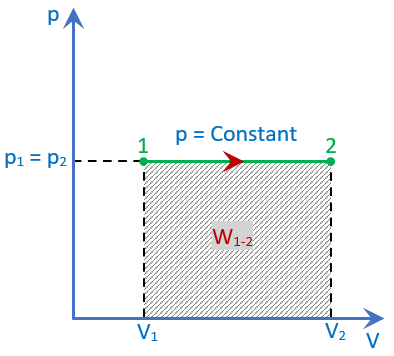It is a thermodynamic process that takes place at constant pressure. The Isobaric is derived from Greek “Iso” and “Baros”, meaning “Equal Pressure”.

In a thermodynamics process as the volume changes, pressure change occurs. To maintian the pressure constant during the process heat is added and removed. When heat is supplied or removed from a thermodynamic system in an isobaric process, as a result work is done. However, there is a change in the system's internal energy. The Isobaric process is also known as the constant pressure process.

Consider a system undergoing Isobaric process from state 1 to state 2 as shown in the figure. The gas system is in thermodynamic equilibrium and is described by the coordinates p1, V1, p2 and V2

And the Workdone for any Quasi static process can be written as,

-1

For isobaric process; As p=C, will be taken out of integral

On integrating, we get

-2

Equation 2, gives Work Done during the Isobaric process.

Isochoric Process [V = Constant]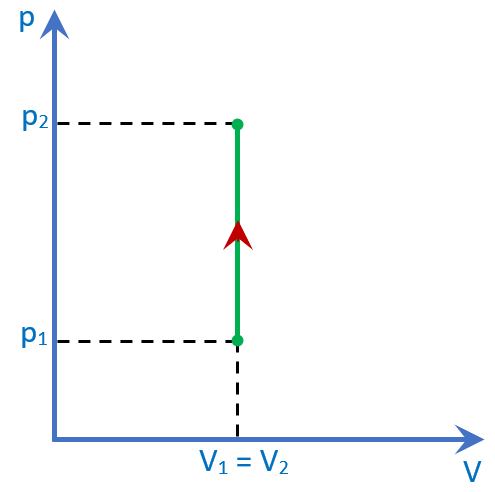An isochoric process, also called a constant volume process, is a thermodynamic process during which the addition or removal of heat when volume is constant. The isochoric process here should be a quasi static process.

When the gas is heated at a constant volume, its temperature and pressure will increase. Since there is no change in its volume, no external work is done by the gas. All the heat supplied is stored in the body of the gas in the form of internal energy.

And the Workdone for any Quasi static process can be written as,

-1

For Isochoric process Volume is constant; V = C

Workdone during Isochoric process is zero as there is no change in volume

Isothermal Process [ pV = Constant]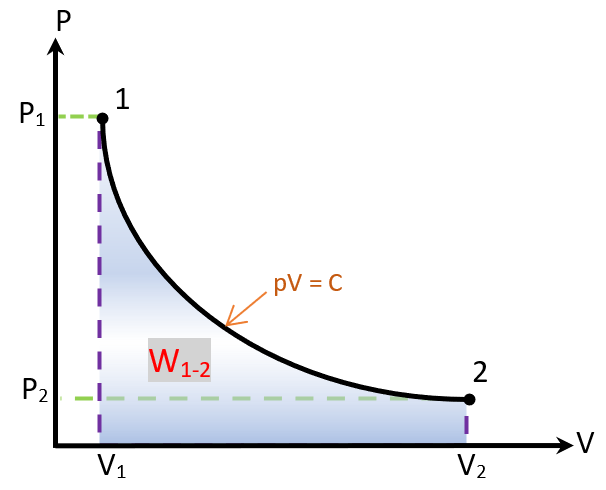Isothermal Process - The process in which the temperature of the working substance remains constant during expansion or compression. It is also called a constant temperature process and sometimes a hyperbolic process.

Hyperbolic Process - is the process where the working substance is heated or expanded in such a way that the product of its pressure and volume remains constant.The curve for such an expansion process is a rectangular hyperbola and hence this is known as hyperbolic expansion. The process is governed by Boyle’s law.

Main Difference between Isothermal and Hyperbolic processes is that the working medium in the hyperbolic process is vapour and the working medium in the Isothermal process is gas.

And the Workdone for any Quasi static process can be written as,

-1

For isothermal process,

we have,; therefore we can rewrite as,

Now replacing,  in equation -1 we get

As  , will be taken out of integral

On integrating, we get

-2

Also ; therefore we can rewrite as,

Now replacing,  in equation -2 we get

-3

Equations 2 and 3, give Work Done during the Isothermal process.

Polytropic Process [ pVn = Constant]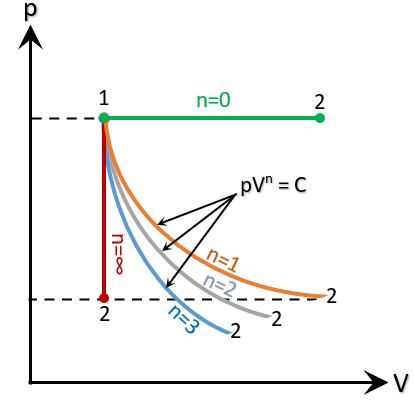A polytropic process is any thermodynamic process that can be expressed by the following equation,

The polytropic process equation describes expansion and compression processes which include heat transfer. The exponent ’n’ is known as the polytropic index and it may take on any value from 0 (Zero) to ∞ (Infinity), depending on the particular process.

There are some special cases of n, which corresponds to particular processes;

The case n = 0; , corresponds to an Isobaric process.
The case n = 1;
,  corresponds to an Isothermal or Hyperbolic process.
The case n =
; ,  corresponds to an Isentropic (adiabatic) process. And the adiabatic index
where -  CP = Heat capacity at constant pressure

CV = Heat capacity at constant volume

The case n ➝ ∞;  V = 0, corresponds to an Isochoric Process.
And the Workdone for any Quasi static process can be written as,

-1

For Polytropic process;

We have, ; therefore we can rewrite as,

Now replacing,  in equation -1 we get

As ; will be taken out of integral

On integrating, we get

Also, we know that

-2

Equations 2, give Work Done during the Polytropic process.

Adiabatic Process [ pVγ = Constant]

v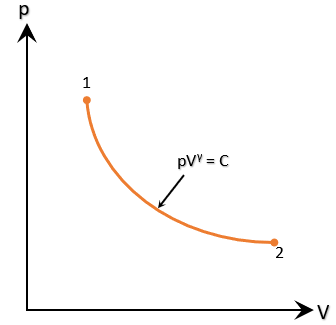An adiabatic process is a thermodynamic process, in which there is no heat transfer in or out of the system from its surroundings neither during expansion nor during compression. In an adiabatic process, work is the only energy transfer taking place.

The adiabatic process can be either reversible or irreversible. The assumption of no heat transfer is very important. Following are the characteristics of adiabatic process;

• The system must be perfectly insulated.

• The process must be carried out instantaneously, so there is not enough time for heat transfer.

And the Workdone for any Quasi static process can be written as,

-1

We have , ; therefore we can rewrite as

Now replacing,  in equation -1 we get

As  ; will be taken out of integral

On integrating, we get

Also, we know that

\ -2

Equations 2, give Work Done during the Adiabatic process.